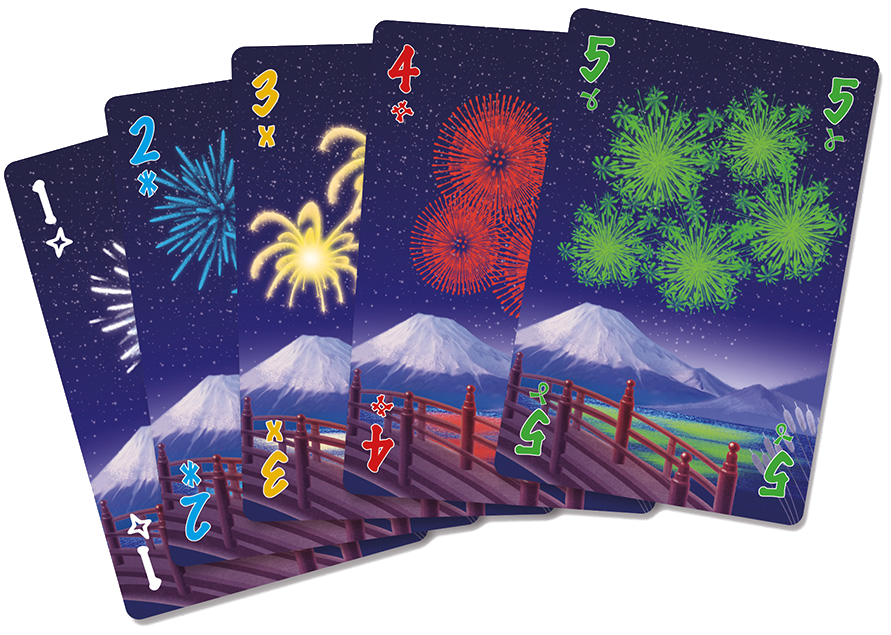# Hanabi

A cooperative card game in which players can see others' cards but not their own. Players exchange hints with the goal of playing the cards in a specific order.## Description

Hanabi is a a cooperative multiplayer card game with imperfect information. An hanabi card $(v,c)$ consists of an integer value $v$ between $1$ an $n$ and of a color $c$ out of $C$ possible. Up to $r$ copies of the same card might appear in an deck consisting of $N \le n \cdot c \cdot h$ cards. Throughout the game, the cards held by one player are only visible by the other players. Additionally, players share a certain number of information tokens.

At the beginning of the game Players draw $h$ cards each. Then, the players play in turns, where each turn consists of one of the following actions:

• Give information by announcing either a color or a number and pointing at all the cards of that color or number in another player’s hand. This action consumes one information token.

• Discard a card from the current player’s hand and replenish one information token.

• Play a card $(v,c)$ from the current player’s hand. This is successful if (i) $v=1$ and no other card of color $c$ has been successfully played, or (ii) the card $(v-1,c)$ has been successfully player but the card $(v,c)$ has not. If this action is not successful the card is discarded. Regardless of whether the action is successful, the player discards the played card and draws a replacement, unless the deck is empty.

The game terminates whenever one of the following conditions holds:

• A set number of cards are unsuccessfully played.

• All cards $(n,c)$ for each color $c$ have been successfully played.

• Each player has played once since the deck became empty.

When the game ends, it is scored by summing the values of the highest-value card of each color that has been successfully played.

In the classical version of the game $n=5$, $c=5$ (white, yellow, green, blue, and red), $h \in {4,5}$, the game ends after $3$ mistakes, and the number of information tokens is $3$.

## Computational complexity

The variant in which there is a unique player with full knowledge over the order of the cards in the deck has been considered in . In this variant the player draws a card and can discard it, store it for later, or play it right away along with any number of stored cards. Up to $h$ cards can be stored, which corresponds to an hand size of $h+1$ in the original game. The goal is to determine whether there is a strategy that (successfully) plays all the cards of values $1,2,…, n$ for each color.

The results for the above variant are as follows :

• Solvable in $O(N(h^2 + hc)c^h n^{h+c-1} )$ time.

• Solvable in $O(N)$ time if $r=1$.

• Solvable in $O(N + n \log h)$ time if $c=1$.

• NP-complete when $h \ge 2$ and $r \ge 2$.

• NP-complete when $h = 1$ and $r \ge 3$.

 J.-F. Baffier, M.-K. Chiu, Y. Diez, M. Korman, V. Mitsou, A. van Renssen, M. Roeloffzen, Y. Uno, “Hanabi is NP-hard, even for cheaters who look at their cards”, Theoretical Computer Science, 2017.

@misc{cog:hanabi,
author = "{CoG contributors}",
title  = "{Hanabi --- Complexity of Games}",
year   = "2022",
url    = "https://www.isnphard.com/i/hanabi/",
note   = "[Online; accessed 2022-06-02]"
}